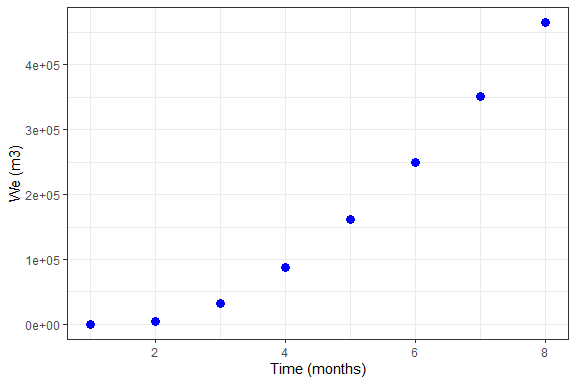# Raquifer

## Raquifer

Raquifer estimates the cumulative water influx into hydrocarbon reservoirs using un-steady and pseudo-steady state modeling approaches. It generates a data frame of cumulative water influx over time for edge-drive and bottom-drive aquifers. Van Everdingen and Hurst un-steady state model for the constant terminal pressure solution predicts the cumulative influx for edge-water drive aquifers with radial flow (Van Everdingen & Hurst, 1949). For the bottom-water drive aquifers with linear/radial flow, the Yildiz-Khosravi un-steady state model for the constant terminal pressure solution is used (Yildiz & Khosravi, 2007). Nabor and Barham linear flow model for the constant terminal pressure solution is used for the edge-water and bottom-water drive aquifers modeling(Nabor & Barham, 1964). For the linear and radial pseudo-steady state flow modeling in aquifers, the Fetkovich method is used (Fetkovich, 1971).

Cumulative water influx predictions are generated by three different functions: aquifer_param(), aquifer_time(), and aquifer_predict().

## aquifer_param() arguments

• input_unit: A unit system for parameters, a character string either ‘SI’ or ‘Field’
• output_unit: A unit system for properties, a character string either ‘SI’ or ‘Field’
• param model: State of flow in the aquifer, a character string either ‘uss’ for the un-steady state flow or ‘pss’ for the pseudo-steady state flow
• flow_type: A character string either ‘radial’ or ‘linear’
• water_drive: A character string either ‘edge’ or ‘bottom’
• phi: Aquifer porosity, a numeric fraction
• perm_h: Aquifer horizontal permeability in ‘md’ in both ‘SI’ and ‘Field’ input unit systems. A NULL value must be used for the combination of ‘uss’, ‘linear’, and ‘bottom’ flow
• perm_v: Aquifer vertical permeability in ‘md’ in both ‘SI’ and ‘Field’ input unit systems. A NULL value must be used for the combination of ‘uss’, ‘linear’, ‘edge’ flow. A NULL value must be used for the combination of ‘uss’, ‘radial’, ‘edge’ flow. A NULL value must be used for the combination of ‘pss’, ‘radial’, ‘edge’ flow.
• h_a: Aquifer height in ‘m’ or ‘ft’ in ‘SI’ and ‘Field’ input unit systems, respectively.
• r_a: Aquifer radius in ‘m’ or ‘ft’ in ‘SI’ and ‘Field’ input unit systems, respectively. A NULL value must be used for the combination of ‘uss’, ‘linear’, ‘edge’ flow. A NULL value must be used for the combination of ‘uss’, ‘linear’, ‘bottom’ flow.
• r_R: Reservoir radius in ‘m’ or ‘ft’ in ‘SI’ and ‘Field’ input unit systems, respectively. A NULL value must be used for the combination of ‘uss’, ‘linear’, ‘edge’ flow. A NULL value must be used for the combination of ‘uss’, ‘linear’, ‘bottom’ flow.
• w_a: Aquifer width in ‘m’ or ‘ft’ in ‘SI’ and ‘Field’ input unit systems, respectively. A NULL value must be used for the combination of ‘uss’, ‘radial’, ‘edge’ flow. A NULL value must be used for the combination of ‘uss’, ‘radial’, ‘bottom’ flow. A NULL value must be used for the combination of ‘pss’, ‘radial’, ‘edge’ flow.
• l_a: Aquifer length in ‘m’ or ‘ft’ in ‘SI’ and ‘Field’ input unit systems, respectively. A NULL value must be used for the combination of ‘uss’, ‘radial’, ‘edge’ flow. A NULL value must be used for the combination of ‘uss’, ‘radial’, ‘bottom’ flow. A NULL value must be used for the combination of ‘pss’, ‘radial’, ‘edge’ flow.
• tetha: Fraction of reservoir encircled by the aquifer, reported in “degrees” in both ‘SI’ and ‘Field’ input unit systems. A NULL value must be used for the combination of ‘uss’, ‘radial’, ‘bottom’ flow. A NULL value must be used for the combination of ‘uss’, ‘linear’, ‘edge’ flow. A NULL value must be used for the combination of ‘uss’, ‘linear’, ‘bottom’ flow.
• mu_water: Water viscosity in ‘mPa.s’ or ‘cp’ in ‘SI’ and ‘Field’ input unit systems, respectively
• c_water: Water compressibility in ‘1/kPa’ or ‘1/psi’ in ‘SI’ and ‘Field’ input unit systems, respectively
• c_rock: Rock compressibility in ‘1/kPa’ or ‘1/psi’ in ‘SI’ and ‘Field’ input unit systems, respectively
• pressure: A numeric vector of pressure data at the boundary of reservoir/aquifer. Must have the same length as the ‘aquifer_time()’ object

## aquifer_time() arguments

• x: A vector or sequence of times/dates.
• unit: A unit system for input vector x.

## aquifer_predict() arguments

• aquifer_lst: A list object of class ‘decline’.
• time_lst: A list object of class ‘time’

## Installation

The Raquifer can be installed from CRAN with:

install.packages("Raquifer")

## Examples

#### Example 1: Un-steady state radial flow, edge-water drive

library(Raquifer)
library(ggplot2)
library(magrittr)

aqu_time <- aquifer_time(x = c(0,0.368,2.439,4.957,7.732,11.926,18.126,30.044) * 365, unit = "day")

parameters <- aquifer_param(input_unit = "Field", output_unit = "Field", model = "uss",
flow_type = "radial", water_drive = "edge", phi = 0.27, perm_h = 64.2,
h_a = 20, r_a = 5 * 14892, r_R = 14892, tetha = 180,
mu_water = 0.485, c_water = 3.88e-6, c_rock = 2e-6,
pressure = c(1640,1600,1400,1200,1000,800,600,400))

aqu_time
#> $t #>  0.000 134.320 890.235 1809.305 2822.180 4352.990 6615.990 #>  10966.060 #> #>$unit
#>  "day"
#>
#> $reference_date #>  "2020-05-12" #> #> attr(,"class") #>  "day" "time" parameters #>$input_unit
#>  "Field"
#>
#> $output_unit #>  "Field" #> #>$model
#>  "veh_rad_edge"
#>
#> $phi #>  0.27 #> #>$perm_h
#>  64.2
#>
#> $h_a #>  20 #> #>$r_a
#>  74460
#>
#> $r_R #>  14892 #> #>$tetha
#>  180
#>
#> $mu_water #>  0.485 #> #>$c_water
#>  3.88e-06
#>
#> $c_rock #>  2e-06 #> #>$pressure
#>  1640 1600 1400 1200 1000  800  600  400
#>
#> attr(,"class")
#>  "veh_rad_edge" "aquifer"

pred_veh <- aquifer_predict(aquifer_lst = parameters, time_lst = aqu_time)

head(pred_veh)
#>         Date Time (days)  We (MMbbl)
#> 1 2020-05-12       0.000  0.00000000
#> 2 2020-09-23     134.320  0.06185335
#> 3 2022-10-19     890.235  1.27831951
#> 4 2025-04-25    1809.305  4.19222802
#> 5 2028-02-02    2822.180  8.46586625
#> 6 2032-04-11    4352.990 15.65627937

pred_veh %>% ggplot(aes(x = Time (days), y = We (MMbbl))) +
geom_point(size = 3, color = "blue") +
theme_bw()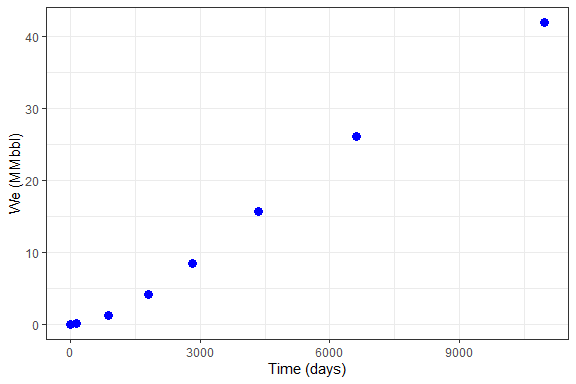#### Example 2: Un-steady state radial flow, bottom-water drive

library(Raquifer)
library(ggplot2)
library(magrittr)

aqu_time <- aquifer_time(x = c(0,0.368,2.439,4.957,7.732,11.926,18.126,30.044) * 365, unit = "day")

parameters <- aquifer_param(input_unit = "Field", output_unit = "Field", model = "uss",
flow_type = "radial", water_drive = "bottom", phi = 0.27, perm_h = 64.2,
perm_v = 64.2, h_a = 20, r_a = 5 * 14892, r_R = 14892,
mu_water = 0.485, c_water = 3.88e-6, c_rock = 2e-6,
pressure = c(1640,1600,1400,1200,1000,800,600,400))

pred_ykh <- aquifer_predict(aquifer_lst = parameters, time_lst = aqu_time)

head(pred_ykh)
#>         Date Time (days) We (MMbbl)
#> 1 2020-05-12       0.000  0.0000000
#> 2 2020-09-23     134.320  0.1600501
#> 3 2022-10-19     890.235  2.5380986
#> 4 2025-04-25    1809.305  8.0646132
#> 5 2028-02-02    2822.180 16.0265710
#> 6 2032-04-11    4352.990 29.3508862

pred_ykh %>% ggplot(aes(x = Time (days), y = We (MMbbl))) +
geom_point(size = 3, color = "blue") +
theme_bw()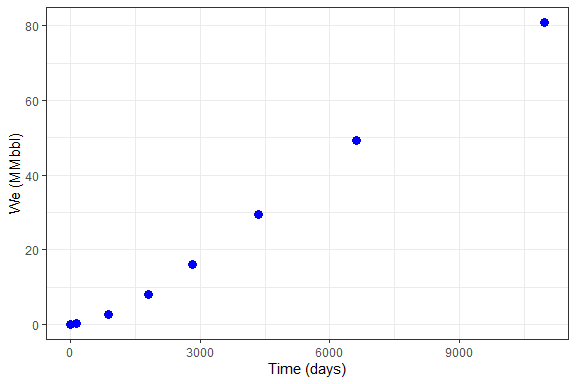#### Example 3: Pseudo-steady state radial flow, edge-water drive

library(Raquifer)
library(ggplot2)
library(magrittr)

aqu_time <- aquifer_time(x = c(0,0.368,2.439,4.957,7.732,11.926,18.126,30.044) * 365, unit = "day")

parameters <- aquifer_param(input_unit = "Field", output_unit = "Field", model = "pss",
flow_type = "radial", water_drive = "edge", phi = 0.27, perm_h = 64.2,
h_a = 20, r_a = 5 * 14892, r_R = 14892, tetha = 180,
mu_water = 0.485, c_water = 3.88e-6, c_rock = 2e-6,
pressure = c(1640,1600,1400,1200,1000,800,600,400))

pred_fetk <- aquifer_predict(aquifer_lst = parameters, time_lst = aqu_time)

head(pred_fetk)
#>         Date Time (days)  We (MMbbl)
#> 1 2020-05-12       0.000  0.00000000
#> 2 2020-09-23     134.320  0.02884514
#> 3 2022-10-19     890.235  1.08324703
#> 4 2025-04-25    1809.305  3.94690549
#> 5 2028-02-02    2822.180  8.44348341
#> 6 2032-04-11    4352.990 16.33925334

pred_fetk %>% ggplot(aes(x = Time (days), y = We (MMbbl))) +
geom_point(size = 3, color = "blue") +
theme_bw()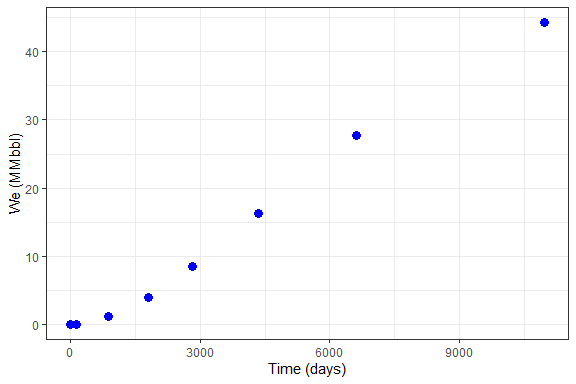#### Example 4: Un-steady state linear flow, edge-water drive

library(Raquifer)
library(ggplot2)
library(magrittr)

aqu_time <- aquifer_time(x = c(0,0.368,2.439,4.957,7.732,11.926,18.126,30.044) * 365, unit = "day")

parameters <- aquifer_param(input_unit = "Field", output_unit = "Field", model = "uss",
flow_type = "linear", water_drive = "edge", phi = 0.27, perm_h = 64.2,
h_a = 20, w_a = 29784, l_a = 161145, mu_water = 0.485, c_water = 3.88e-6,
c_rock = 2e-6, pressure = c(1640,1600,1400,1200,1000,800,600,400))

pred_nb_01 <- aquifer_predict(aquifer_lst = parameters, time_lst = aqu_time)

head(pred_nb_01)
#>         Date Time (days) We (MMbbl)
#> 1 2020-05-12       0.000 0.00000000
#> 2 2020-09-23     134.320 0.03199772
#> 3 2022-10-19     890.235 0.53782163
#> 4 2025-04-25    1809.305 1.63239439
#> 5 2028-02-02    2822.180 3.09768169
#> 6 2032-04-11    4352.990 5.35540873

pred_nb_01 %>% ggplot(aes(x = Time (days), y = We (MMbbl))) +
geom_point(size = 3, color = "blue") +
theme_bw()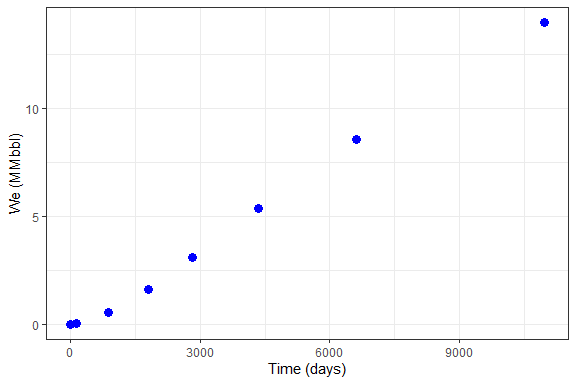#### Example 5: Un-steady state linear flow, bottom-water drive

library(Raquifer)
library(ggplot2)
library(magrittr)

aqu_time <- aquifer_time(x = c(0,0.368,2.439,4.957,7.732,11.926,18.126,30.044) * 365, unit = "day")

parameters <- aquifer_param(input_unit = "Field", output_unit = "Field", model = "uss",
flow_type = "linear", water_drive = "bottom", phi = 0.27, perm_v = 64.2,
h_a = 20, w_a = 29784, l_a = 161145, mu_water = 0.485, c_water = 3.88e-6,
c_rock = 2e-6, pressure = c(1640,1600,1400,1200,1000,800,600,400))

pred_nb_02 <- aquifer_predict(aquifer_lst = parameters, time_lst = aqu_time)

head(pred_nb_02)
#>         Date Time (days) We (MMbbl)
#> 1 2020-05-12       0.000  0.0000000
#> 2 2020-09-23     134.320  0.5428142
#> 3 2022-10-19     890.235  3.7996992
#> 4 2025-04-25    1809.305  9.2278409
#> 5 2028-02-02    2822.180 14.6559827
#> 6 2032-04-11    4352.990 20.0841244

pred_nb_02 %>% ggplot(aes(x = Time (days), y = We (MMbbl))) +
geom_point(size = 3, color = "blue") +
theme_bw()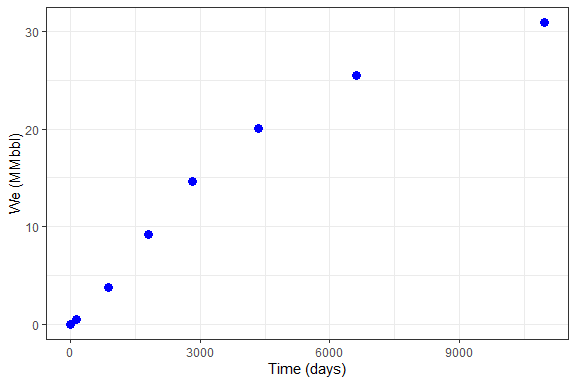#### Example 6: Pseudo-steady state linear flow, edge-water drive

library(Raquifer)
library(ggplot2)
library(magrittr)

aqu_time <- aquifer_time(x = c(0,0.368,2.439,4.957,7.732,11.926,18.126,30.044) * 365, unit = "day")

parameters <- aquifer_param(input_unit = "Field", output_unit = "Field", model = "pss",
flow_type = "linear", water_drive = "edge", phi = 0.27, perm_h = 64.2,
h_a = 20, w_a = 29784, l_a = 161145, mu_water = 0.485, c_water = 3.88e-6,
c_rock = 2e-6, pressure = c(1640,1600,1400,1200,1000,800,600,400))

parameters
#> $input_unit #>  "Field" #> #>$output_unit
#>  "Field"
#>
#> $model #>  "fetk_lin_edge" #> #>$phi
#>  0.27
#>
#> $perm_h #>  64.2 #> #>$h_a
#>  20
#>
#> $w_a #>  29784 #> #>$l_a
#>  161145
#>
#> $mu_water #>  0.485 #> #>$c_water
#>  3.88e-06
#>
#> $c_rock #>  2e-06 #> #>$pressure
#>  1640 1600 1400 1200 1000  800  600  400
#>
#> attr(,"class")
#>  "fetk_lin_edge" "aquifer"

pred_fetk_02 <- aquifer_predict(aquifer_lst = parameters, time_lst = aqu_time)

head(pred_fetk_02)
#>         Date Time (days)  We (MMbbl)
#> 1 2020-05-12       0.000 0.000000000
#> 2 2020-09-23     134.320 0.004426176
#> 3 2022-10-19     890.235 0.175334148
#> 4 2025-04-25    1809.305 0.668533598
#> 5 2028-02-02    2822.180 1.506005132
#> 6 2032-04-11    4352.990 3.161133684

pred_fetk_02 %>% ggplot(aes(x = Time (days), y = We (MMbbl))) +
geom_point(size = 3, color = "blue") +
theme_bw()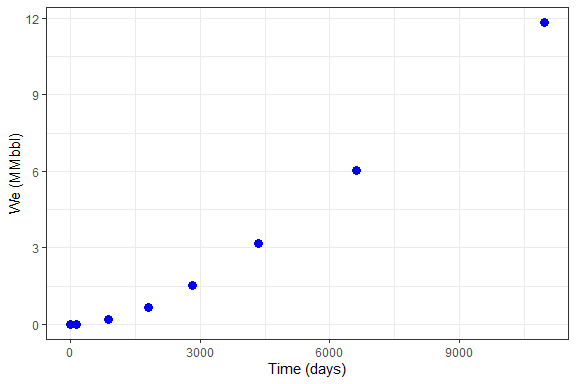#### Example 7: Pseudo-steady state linear flow, bottom-water drive

library(Raquifer)
library(ggplot2)
library(magrittr)

aqu_time <- aquifer_time(x = c(0,0.368,2.439,4.957,7.732,11.926,18.126,30.044) * 365, unit = "day")

parameters <- aquifer_param(input_unit = "Field", output_unit = "Field", model = "pss",
flow_type = "linear", water_drive = "bottom", phi = 0.27, perm_v = 64.2,
h_a = 20, w_a = 29784, l_a = 161145, mu_water = 0.485, c_water = 3.88e-6,
c_rock = 2e-6, pressure = c(1640,1600,1400,1200,1000,800,600,400))

pred_fetk_03 <- aquifer_predict(aquifer_lst = parameters, time_lst = aqu_time)

head(pred_fetk_03)
#>         Date Time (days) We (MMbbl)
#> 1 2020-05-12       0.000  0.0000000
#> 2 2020-09-23     134.320  0.5428142
#> 3 2022-10-19     890.235  3.7996992
#> 4 2025-04-25    1809.305  9.2278409
#> 5 2028-02-02    2822.180 14.6559827
#> 6 2032-04-11    4352.990 20.0841244

pred_fetk_03 %>% ggplot(aes(x = Time (days), y = We (MMbbl))) +
geom_point(size = 3, color = "blue") +
theme_bw()#### Example 8: Un-steady state radial flow, edge-water drive

library(Raquifer)
library(ggplot2)
library(magrittr)

aqu_time <- aquifer_time(x = seq(as.Date("2020/1/1"), by = "year", length.out = 8), unit = "date")

parameters <- aquifer_param(input_unit = "Field", output_unit = "SI", model = "uss",
flow_type = "radial", water_drive = "edge", phi = 0.27, perm_h = 64.2,
h_a = 20, r_a = 5 * 14892, r_R = 14892, tetha = 180,
mu_water = 0.485, c_water = 3.88e-6, c_rock = 2e-6,
pressure = c(1640,1600,1400,1200,1000,800,600,400))

aqu_time
#> $t #>  0 366 731 1096 1461 1827 2192 2557 #> #>$unit
#>  "date"
#>
#> $reference_date #>  "2020-01-01" #> #> attr(,"class") #>  "day" "time" parameters #>$input_unit
#>  "Field"
#>
#> $output_unit #>  "SI" #> #>$model
#>  "veh_rad_edge"
#>
#> $phi #>  0.27 #> #>$perm_h
#>  64.2
#>
#> $h_a #>  20 #> #>$r_a
#>  74460
#>
#> $r_R #>  14892 #> #>$tetha
#>  180
#>
#> $mu_water #>  0.485 #> #>$c_water
#>  3.88e-06
#>
#> $c_rock #>  2e-06 #> #>$pressure
#>  1640 1600 1400 1200 1000  800  600  400
#>
#> attr(,"class")
#>  "veh_rad_edge" "aquifer"

pred_veh <- aquifer_predict(aquifer_lst = parameters, time_lst = aqu_time)

head(pred_veh)
#>         Date Time (days)   We (m3)
#> 1 2020-01-01           0       0.0
#> 2 2021-01-01         366   18021.0
#> 3 2022-01-01         731  135900.7
#> 4 2023-01-01        1096  383984.4
#> 5 2024-01-01        1461  722190.1
#> 6 2025-01-01        1827 1141218.1

pred_veh %>% ggplot(aes(x = Time (days), y = We (m3))) +
geom_point(size = 3, color = "blue") +
theme_bw()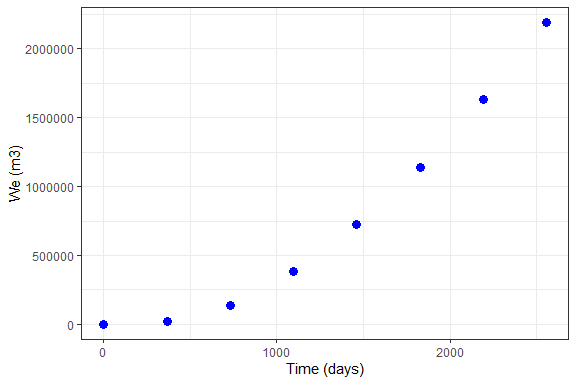#### Example 8: Un-steady state radial flow, edge-water drive

library(Raquifer)
library(ggplot2)
library(magrittr)

aqu_time <- aquifer_time(x = 1:8, unit = "month")

parameters <- aquifer_param(input_unit = "Field", output_unit = "SI", model = "uss",
flow_type = "radial", water_drive = "edge", phi = 0.27, perm_h = 64.2,
h_a = 20, r_a = 5 * 14892, r_R = 14892, tetha = 180,
mu_water = 0.485, c_water = 3.88e-6, c_rock = 2e-6,
pressure = c(1640,1600,1400,1200,1000,800,600,400))

aqu_time
#> $t #>  1 2 3 4 5 6 7 8 #> #>$unit
#>  "month"
#>
#> $reference_date #>  "2020-05-12" #> #> attr(,"class") #>  "month" "time" parameters #>$input_unit
#>  "Field"
#>
#> $output_unit #>  "SI" #> #>$model
#>  "veh_rad_edge"
#>
#> $phi #>  0.27 #> #>$perm_h
#>  64.2
#>
#> $h_a #>  20 #> #>$r_a
#>  74460
#>
#> $r_R #>  14892 #> #>$tetha
#>  180
#>
#> $mu_water #>  0.485 #> #>$c_water
#>  3.88e-06
#>
#> $c_rock #>  2e-06 #> #>$pressure
#>  1640 1600 1400 1200 1000  800  600  400
#>
#> attr(,"class")
#>  "veh_rad_edge" "aquifer"

pred_veh <- aquifer_predict(aquifer_lst = parameters, time_lst = aqu_time)

head(pred_veh)
#>         Date Time (months)    We (m3)
#> 1 2020-06-11             1      0.000
#> 2 2020-07-11             2   4236.423
#> 3 2020-08-11             3  31650.466
#> 4 2020-09-10             4  87609.327
#> 5 2020-10-11             5 161084.687
#> 6 2020-11-10             6 249479.619

pred_veh %>% ggplot(aes(x = Time (months), y = We (m3))) +
geom_point(size = 3, color = "blue") +
theme_bw()`